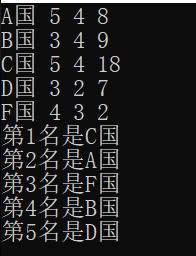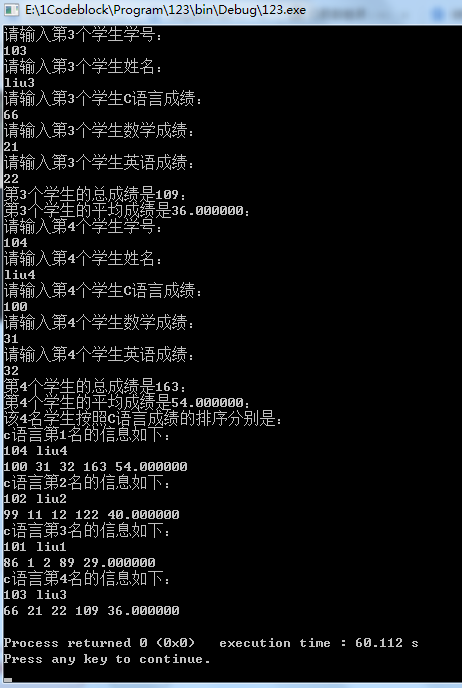• sort函数对结构体数组排序
2021-03-24 19:19:28

sort函数对结构体数组排序

#include<iostream>
#include<algorithm>
using namespace std;
struct st
{
int a1;
int b1;
}arr;
//首先 &是取地址运算符   在cmp中 把结构体的地址赋值给了 x ,y ;
//x对应sort函数的第一个参数，y 对应sort函数的第二个参数
// x.b1 与  y.b1 就确定了  排序的元素和大小顺序
bool  cmp(const st &x, const st &y)
{
return x.b1<y.b1;//从小到大排<，若要从大到小排则>
}
int main()
{
int i;
for (i=0;i<3;i++)
cin>>arr[i].a1>>arr[i].b1;//对结构体进行输入
sort(arr,arr+3,cmp);
for (i=0;i<3;i++)
cout<<arr[i].a1<<" "<<arr[i].b1<<endl;
}

更多相关内容
• MatLab 函数“orderfields”的递归版本。... 与仅顶级字段排序的“orderfields”相反，函数“OrderAllFields”在其所有级别上对结构体数组的字段进行排序（从而也传递具有元胞数组结构而不是结构体数组结构的级别）。matlab
• 对结构体数组进行排序。 题目描述： struct Person{ 　int no; 　int age; 　int height; } 实现sort方法对结构体数组进行排序。 void sort(Person * array,int n); 根据no从小到大排序；如果no相同则根据age排序...

题目描述：

对结构体数组进行排序。

题目描述：
struct Person{
int no;
int age;
int height;
}
实现sort方法对结构体数组进行排序。
void sort(Person * array,int n);

根据no从小到大排序；如果no相同则根据age排序；如果age相同，则根据height排序。

注意：
遇到异常情况，输出"error"；否则不要随意输出，会视为错误。

参考代码：

#include<stdio.h>
struct Person{
int no;
int age;
int height;
};
void sort(struct Person * array,int n)
{
if(array==NULL||n<=0)
{
printf("error");
return;
}
int ex,i,j;
for(i=0;i<n-1;i++)
{
for(j=0;j<n-1-i;j++)
{
if(array[j].no>array[j+1].no||(array[j].no==array[j+1].no&&array[j].age>array[j+1].age)||(array[j].no==array[j+1].no&&array[j].age==array[j+1].age&&array[j].height>array[j+1].height))
{
ex=array[j].no;
array[j].no=array[j+1].no;
array[j+1].no=ex;
ex=array[j].age;
array[j].age=array[j+1].age;
array[j+1].age=ex;
ex=array[j].height;
array[j].height=array[j+1].height;
array[j+1].height=ex;
}
}
}
return;
}
int main()
{
int n,i;
struct Person a;
scanf("%d",&n);
for(i=0;i<n;i++)
scanf("%d %d %d",&a[i].no,&a[i].age,&a[i].height);
sort(a,n);
for(i=0;i<n;i++)
printf("%d %d %d\n",a[i].no,a[i].age,a[i].height);
return 0;
}

讲解：

结构体swap的升级版（多了个循环）。

# 求实求真，大气大为。

展开全文c语言
• 生活，我们经常会遇到排序问题，像班级成绩排序、奥运奖牌排名等。而像这些往往会涉及到多变量。就不能把它处理成简单地一维数组排序，需sort函数和自定义函数结合使用。 ...

生活中，我们经常会遇到排序问题，像班级成绩排序、奥运奖牌排名等。而像这些往往会涉及到多变量。就不能把它处理成简单地一维数组排序，需sort函数和自定义函数结合使用。

 金牌 银牌 铜牌 A国 5 4 8 B国 3 4 9 C国 5 4 18 D国 3 2 7 F国 4 3 2

要求对上表格中的5个国家进行排序，排序等级金牌>银牌>铜牌

#include<iostream>
#include<cstring>
#include<algorithm>
using namespace std;
struct award{
string name;
int gold;
int silver;
int bronze;
} cnt;
bool fun(award x,award y){
if (x.gold != y.gold)
return x.gold > y.gold;
else if (x.silver != y.silver)
return x.silver > y.silver;
else
return x.bronze > y.bronze;
}
int main()
{
for (int i = 0; i < 5; i++)
cin >> cnt[i].name >> cnt[i].gold >> cnt[i].silver >> cnt[i].bronze;
sort(cnt,cnt+5,fun);
for (int i = 0; i < 5; i++)
cout << "第"<< i+1 << "名是" << cnt[i].name << endl;
return 0;
}展开全文/*
*作 者：HH
*完成日期：2018年8月17日
*版本号：v1.0
*
*问题描述:输入结构体数组，并根据结构体中的某一数据项对整个结构体数组进行排序
*输入描述：；
*程序输出：
*/

#include<stdio.h>
#include<string.h>

struct student
{
int num;
char name;
int cpp;
int math;
int english;
double average;
};
struct student per;
struct student test;

int main()
{
int n,m;
printf("请输入需要统计信息的学生个数：\n");
scanf("%d",&m);
printf("请依次输入该%d名学生的信息\n",m);
for(n=0;n<m;n++)
{
printf("请输入第%d个学生学号：\n",n+1);
scanf("%d",&per[n].num);
printf("请输入第%d个学生姓名：\n",n+1);
scanf("%s",&per[n].name);
printf("请输入第%d个学生C语言成绩：\n",n+1);
scanf("%d",&per[n].cpp);
printf("请输入第%d个学生数学成绩：\n",n+1);
scanf("%d",&per[n].math);
printf("请输入第%d个学生英语成绩：\n",n+1);
scanf("%d",&per[n].english);
printf("第%d个学生的平均成绩是%f：\n",n+1,per[n].average);
}
int i,j;
for(i=0;i<m;i++)
{
for(j=0;j<m-i-1;j++)//此处很重要
{
if(per[j].cpp<per[j+1].cpp)//根据单一数据项，对整个结构体进行排序
{
test=per[j+1];//结构体允许整体赋值
per[j+1]=per[j];
per[j]=test;
}
}
}
printf("该%d名学生按照C语言成绩的排序分别是：\n",m);
for(i=0;i<m;i++)
{
printf("c语言第%d名的信息如下：\n",i+1);
printf("%d %s\n",per[i].num,per[i].name);
}
return 0;
}展开全文• 用模拟的qsort进行解释结构体数组排序 typedef struct Stu{ char name; char sex; }stu; void Swap(void *_x, void *_y,int size) { char *x = (char *)_x; char *y = (char *)_y; char temp = 0; ...qsort 结构体数组 c语言
• 匿名用户 1级 2014-08-31 回答 ... 追问： 代码是纯c的，编译通过能运行，不排序输出数组每个元素的字符串正常。 以上您的代码没有任何问题。问题出在我这样分配不对，修改后正确了。 非常感谢这么晚了，帮我解答问题。
• 案例描述：使用一个学生结构体，将班级的学生按照成绩...2、创建一个学生结构体数组，并赋值 Student sdu = { //学生的信息 {18,95,"Student_A"}, {18,65,"Student_B"}, {18,78,"Student_C"}, {18,62,"Sc++
• 1_对结构体数组进行排序 通过课程进入 题 号: 41 1_对结构体数组进行排序 语言要求： C++ 对结构体数组进行排序。 题目描述： struct Person{ 　int no; 　int age; 　int height; } 实现sort方法对结构体数组进行...c语言
• ## （C语言）对结构体数组进行排序

万次阅读 多人点赞 2019-11-29 16:40:29
对结构体数组进行排序 题目：对结构体数组进行排序。 题目描述： struct Person{ 　int no; 　int age; 　int height; } 实现sort方法对结构体数组进行排序。 void sort(Person * array,int n); 根据no从小到大排序...C语言
• 一、给结构体数组排序 package main import ( "fmt" "math/rand" "sort" ) //声明一个结构体 type Hero struct { Name string Age int } //声明一个结构体切片 type HeroSlice []Hero //实现Interface接口 ...golang 开发语言 后端
• 但是对结构体数组排序一直没搞懂。#include#includestruct liebiao{int hao;char name;float fen1;float fen2;float fen3;float pingjun;}data,*p=data;int pingjun();void paiming();...
• 数组排序(从小到大,从大到小) 结构体排序(数字参数从大到小…字符串为参数 字典序…) 代码示例:(直接复制运行对比结果看源码) /* sort 排序函数 可以调用 自定义 参数cmp sort(a,a+10,cmp); */ #include<...
• 在按照某一成分对结构体进行排序时，其基本思路和数组排序一样，可以利用指针进行简化，也可以直接利用结构体名进行排序，如下实例，是利用平均分数学生这一结构体进行排序的1、定义结构体struct student//定义...C语言
• C语言，使用qsort函数自定义结构体数组进行排序c语言
• 1.按照结构体数组的某一项排序，那么一个结构体包含的其他元素仍保持不变。也就是说只能选择其中一项作为指标进行排序，相应的其他值对应不变化。 2.排序后仍然是它们的组合，只是顺序变了而已 3.如果希望升序排序,...
• Go语言的sort包帮我们实现了任一类型的数组进行排序。 对于将要排序数组类型只需要我们实现下面三种方法： type Interface interface { Len() int // 数组长度 Less(i, j int) bool //两个元素的大小比较 ...golang 算法
• #include "stdio.h" #include "string.h" struct Student{ int num; char name; float score; }; int main(){ struct Student stu = {{1,"gan",90},{2,"li",98},{3,"zhao",80},{4,"chen",86...
• 要注意强制转换 #include <stdio.h> #include <stdlib.h> typedef struct{ int num; char name; float chinese; float math; float english; }Stu,*pStu;...void arrPrint...
• C语言第11题：结构体数组的定义与使用 + 结构体数组排序C语言第11题：结构体数组的定义与使用 + 结构体数组排序注意：一下赋值是不能使用的但是在结构体是可以直接进行赋值操作的st = st;printf(“姓名 = %s...
• 对结构体数组里面的内容进行排序 #include"stdio.h" #include"string.h" #define TYPE student typedef struct{ char name; char school; int age; }student; typedef int(*pFun)(TYPE, TYPE);//把pFun...c语言 c++
• /******将结构体数组元素按年龄排序***********/ /*******************************************/ #include<iostream> using namespace std; #include<string> struct hero { string name; int age; ...
• 先按照课程编号从小到大排序，再按照成绩从高到底的顺序进行排序.数据结构
• 结构体中某个成员进行排序，下面代码以成员b1为升序的排序 代码： #include #include using namespace std; struct st { int a1; int b1; }arr; bool cmp(const st &x, const st &y) { return x.b1 } ...
• 分析：本题的突破点在冒泡法对结构体数组排序，其实同整型数组排序一样。首先看普通的冒泡 排序： 代码如下： #include <stdio.h> #define N 5 struct Student{ char name; long stuNo; ...
• 方法一：结构体内重载 #include <iostream> #include<stdio.h> #include <string.h> #include <algorithm> #include <vector> #include <...const int maxn = 1...C++ sort
• #include<stdio.h> #define N 3 int main() { struct Book{ char num; char name;... char writer;... scanf("%s%s%s%f",books[i].num,books[i].name,books[i].writer,&...c语言 开发语言
• 调用：#include<algorithm> sort默认从小到大排序。 从大到小排序： bool cmp(int x,int y){ return x>...对结构体数组排序： struct node{ int name; int data; }a; bool cmp(no算法 数据结构
• ## 结构体和结构体数组

千次阅读 多人点赞 2020-03-14 11:14:21
一组数组异质的数组，但自成整体的数据 结构体变量的定义方法: 先声明结构体类型，再定义变量类型。 声明结构体变量时不分配空间，在定义结构体变量时才分配内存空间。 例1 学校体育考试，Tom得了一个不错......